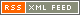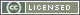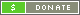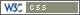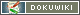# Using The HP3048 Noise Measurement Test Set

There was a fairly long thread on Time-Nuts about how to use the HP 3048 to measure spurs. Here are some of the responses:

Martyn first wrote:

To test the 3048A accuracy I inject a spur at a known level using a
directional coupler (similar to the single-sided spur calibration method).

I confirm the spur level on the spectrum analyzer. However the 3048A
always says the spur is 6 dB lower than it actually is (6 dB plus/minus 0.5 dB).

So who is right? The spectrum analyser or the HP3048A.

Perhaps they are both right:

• The spectrum analyzer is displaying the power spectrum of the RF signal
• The 3048A is displaying the power spectrum of the demodulated phase of the RF signal

Questions:

• Are you asking the 3048A to plot L(f) or S(f) ?
• As best I recall, when you ask it to plot L(f), it takes the actual measurement data, which is S(f), and then subtracts 3dB for the noise floor portions of the plot, using the small angle assumption L(f) = S(f) / 2
• I don't remember how it treats the spurs between plot modes, give it a whirl and see how the displayed noise floor and spurious change when you switch between plots of L(f) and S(f)

Have fun, Brian

P.S. I shall not attempt to add to the coherent/non-coherent sideband discussion, but IIRC hard limiting a signal having a small SSB spur (stripping the AM component and leaving just PM) will create a pair of PM sidebands that are 6 dB down from the original spur level as viewed on a spectrum analyzer.

Martyn wrote:

I did do a plot selecting Phase Modulation Spectral Density (dB/Hz) on
the graph instead of dBc/Hz. Here the spurs were 3 dB lower!!!

To make sure I understand your results, here's how I would summarize your measurements, inserting some made-up numbers for observed spur and noise floor levels:

RF analyzer directly measuring 10 MHz signal :

• spur at 10.000100 Hz is @ -50 dBc

3048A L(f) measuring coherently demodulated phase:

• spur at 100 hz is @ -56 dBc
• noise floor is -160 dBc/Hz at 5 MHz offset

3048A Sphi(f) measuring coherently demodulated phase:

• spur at 100 hz is @ -53 dB
• noise floor is -157 dB/Hz at 5 MHz offset

If this is what you are seeing for relative changes in level for the different measurements, I think the 3048A is working just fine.

Here's another experiment to try :

• change spur to an offset of 500 kHz @ -50 dBm this moves the spur into broadband analyzer territory
• re-run your L(f) and S(f) plots at this offset I would expect the same 6 dB, 3dB spur results for L(f), S(f)
• change the measurement setup BW to zero Hz instead of 1 Hz, and measure Sphi(f) again

This forces the 3048A to NOT apply bandwidth corrections to the collected data, so it will display a segmented-looking trace with increasing floor offset across each band break

Brian

 It's been about 7 years since I've actually pushed the buttons on a 3048A, so my recollection of setup and S/W behavior might be a bit fuzzy